Mobile QR Code1. (Dept. of Electronic and Electrical Eng., Ewha Womans University)

Bootstrap, CMOS, fully differential, mirrored-cascode, TIA

## I. INTRODUCTION

Recently, LiDAR(Light Detection And Ranging) systems have been paid a great deal of attention in the applications of range measurements, object recognition, near-field image processing, unmanned autonomous vehicles, etc. Especially, it is expected that they would be utilized in more various fields in near future, because of a number of advantages such as fast lock-on time, small beam spread, and decelerating vehicle detection ability when compared to conventional RF radar systems (1).

Fig. 1 shows a typical LiDAR system that transmits a short optical pulse from the laser source and receives the reflected optical pulses from a target onto the input optical detector(typ. Avalanche photodiodes and PIN photodiodes). Then, the front-end CMOS integrated circuit successfully processes the incoming weak signals, thereby enabling to recognize the position and the shape of a target located in few tens of meter range (2).

In general, optical detectors yield very small output currents. Therefore, it mandates a transimpedance amplifier(TIA) that converts the weak photocurrents into voltage signals of sufficient magnitude for the following signal processing. Also, the optimization of high transimpedance gain and low noise characteristics is required at the receiving end. However, the bandwidth and sensitivity performance of an optical receiver is significantly deteriorated owing to the notorious photodiode capacitance. In addition, a single-ended circuit configuration is typically vulnerable to common-mode noises including power supply noise and crosstalk noise generated from silicon substrate. Hence, a fully differential structure even is desirable from the input stage to improve common-mode rejection ratio, e.g., power supply rejection ratio(PSRR) (3).

This paper presents a novel fully differential TIA by exploiting a mirrored-cascode circuit to a conventional voltage-mode cascode TIA for better sensitivity than a current-mode TIA. Besides, bootstrapping circuit technique is applied to reduce the significant direct effect of the photodiode capacitance on bandwidth and noise characteristics.

## II. Proposed BFD-TIA

Fig. 2 shows the block diagram of the proposed bootstrapped fully differential TIA(BFD-TIA), where the mirrored-cascode(MC) input stage incorporates a voltage-mode cascode configuration with its mirrored cascode circuit to provide differential outputs, hence leading to less susceptibility to common-mode noises. Then, it is followed by a differential post-amplifier(PA) and a current-mode logic output buffer(OB) to obtain fully differential outputs.

Also, bootstrapping circuit technique is exploited in the MC input stage to reduce the direct effect of the photodiode capacitance(C$_{\mathrm{pd}}$) on the degradation of bandwidth and noise performance. In addition, DC offset current cancellation(DCC) is employed at the input node to remove DC offset currents.

### 1. Mirrored-cascode Input Stage

Fig. 3 shows the schematic diagram of the MC input stage. First, the incoming current signals from the photodiode generates the negative output(OUT$_{\mathrm{N}}$) through the cascode circuit with a feedback resistor(R$_{\mathrm{F1}}$). Therein, the drain node of M$_{1}$ provides the 180$^{\mathrm{o}}$ inverted voltage signal(v$_{1}$) with the same magnitude as that of the input node voltage(v$_{\mathrm{in}}$). Then, this inverted voltage(v$_{1}$) passes through an AC-coupling capacitor(C$_{\mathrm{c}}$), appears as the input signal of the MC differential stage, and finally generates the positive output(OUT$_{\mathrm{P}}$) (3). Another capacitor(C$_{1}$) is added to the gate of M$_{2}$ for symmetry at high frequencies to acquire fully differential signaling.

Fig. 3. Schematic diagram of the proposed MC input stage.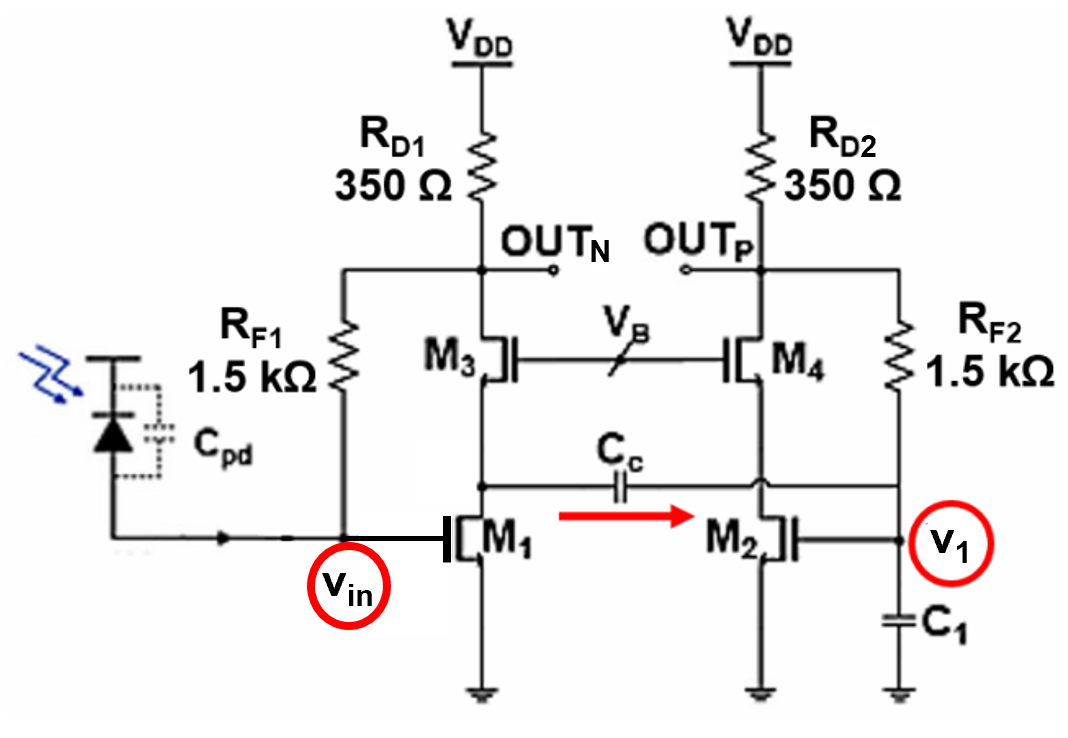According to small signal analysis, the inverted voltage(v$_{1}$) is given by,

##### (1)
\begin{align} \mathrm{\text{v}}_{1}&\cong - g_{m1}\times \left(\left.r_{o1}\right\| \frac{1}{g_{m3}}\right)v_{in}\\ &\cong - \left(g_{m1}\times \frac{1}{g_{m3}}\right)v_{in}\cong ~ - v_{in} \end{align}

The input resistance(R$_{\mathrm{in}}$) and the differential transimpedance gain of the MC input stage are given by,

##### (2)
\begin{align} R_{in}&=~ \frac{v_{in}}{i_{pd}}=\frac{R_{F1}}{1+g_{m1}R_{D1}} \end{align}

##### (3)
\begin{align} \frac{v_{OU{T_{N}}}}{i_{pd}}&=\left(\frac{v_{in}}{i_{pd}}\right)\left(\frac{v_{OU{T_{N}}}}{v_{in}}\right)=- \frac{g_{m1}R_{D1}R_{F1}}{1+g_{m1}R_{D1}}\cong - R_{F1}\\ \frac{v_{OU{T_{P}}}}{i_{pd}}&=\left(\frac{v_{1}}{i_{pd}}\right)\left(\frac{v_{OU{T_{P}}}}{v_{1}}\right)=\frac{g_{m2}R_{D2}R_{F1}}{1+g_{m1}R_{D1}}\cong ~ R_{F1} \end{align}

where it is assumed that g$_{\mathrm{m1}}$ = g$_{\mathrm{m2}}$ and R$_{\mathrm{D1}}$ = R$_{\mathrm{D2}}$.

Therefore, it is clearly seen that the proposed MC input stage provides the same differential transimpedance gain, resulting in a potential to be less sensitive to common-mode noises.

Meanwhile, the equivalent noise current spectral density is given by,

##### (4)
\begin{align} \overline{i_{eq}^{2}}&=\frac{4kT}{R_{F1}}+\omega ^{2}\left(C_{pd}+C_{in1}\right)^{2}\times \left[\frac{4kT}{g_{m1}}+\frac{4kT}{g_{m1}^{2}R_{D1}}\\ ~ ~ ~ ~ ~ ~ ~ ~ +\frac{4kT}{g_{m1}^{2}R_{F2}}+~ ~ \frac{4kT}{g_{m1}^{2}g_{m1}R_{F2}^{2}}+\frac{4kT}{g_{m1}^{2}g_{m2}^{2}R_{F2}^{2}R_{2}}\right]\\ &\cong \frac{4kT}{R_{F1}}+\frac{4kT}{g_{m1}}\times \omega ^{2}\left(C_{pd}+C_{gs1}\right)^{2} \end{align}

Hence, the transconductance(g$_{\mathrm{m1}}$) of M1 should be increased to reduce the noise. Yet, it is noted that either the increase of bias current may lead to large power consumption, or the increase of aspect ratio may enlarge chip area.

Also, the bandwidth of the proposed MC input stage is given by,

##### (5)
\begin{align} f_{3dB}\cong \frac{1+g_{m1}R_{D1}}{R_{f1}\left(C_{pd}+C_{in1}\right)} \end{align}

where it is clearly seen that the bandwidth is inversely proportional to the photodiode capacitance.

Fig. 4(a) depicts the simulated frequency response of the proposed BFD-TIA, showing 86-dB${Ω}$ trans-impedance gain, 373-MHz bandwidth, 7.9-pA/sqrt(Hz) noise current spectral density, -23-dB common-mode noise rejection ratio, and 20-mW power consumption. With the bootstrapping circuit, the BFD-TIA achieves 1.38 times wider bandwidth of 516 MHz and lower noise current spectral density of 7.5 pA/sqrt(Hz). Fig. 4(b) also depicts the simulated eye-diagrams of the BFD-TIA for 2$^{31}$-1 PRBS, where it is clearly seen that two identical eyes are obtained by using the MC input stage.

Fig. 4. Simulation results of the BFD-TIA: (a) frequency response, (b) differential eye-diagrams for 20 ${μ}$A$_{\mathrm{pp}}$ 2$^{31}$-1 PRBS.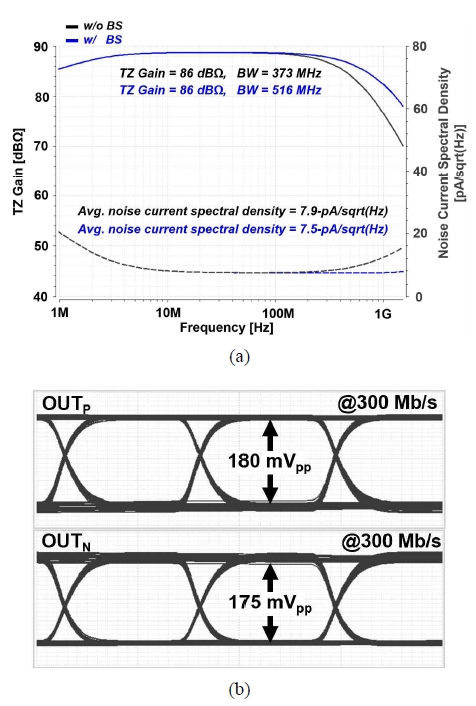### 2. Bootstrapping Stage

Typically, the bandwidth and noise performance of a voltage-mode TIA is greatly deteriorated because of the photodiode capacitance. In this work, we apply the bootstrapping technique in the MC input stage, thereby significantly reducing the effect of the notorious photodiode capacitance.

Fig. 5 shows the schematic diagram of the proposed bootstrapping stage(BS), where the drain-node voltage(v$_{1}$ = -v$_{\mathrm{in}}$) of M$_{1}$ in the MC input stage is utilized as the input signal of the bootstrapping circuit. The BS generates the output signal(v$_{\mathrm{pd}}$) which is equal to v$_{\mathrm{in}}$ by cascading a PMOS source-follower and an NMOS common-source amplifier to provide the voltage gain of -1. Therefore, the AC voltage at the cathode of the photodiode is equal to the input voltage(v$_{\mathrm{in}}$) of the BFD-TIA. Certainly, a slight delay mismatch occurs across the photodiode. Yet, the AC voltage signals across the photodiode are applied with the same magnitude, so that the influence of the photodiode capacitance upon the bandwidth and the noise performance can be greatly reduced.

Fig. 6 shows the transient simulation results of the BS circuit, where it is clearly seen that the input voltage(v$_{\mathrm{in}}$ = 12 mV$_{\mathrm{pp}}$) of the BFD-TIA is almost the same as that of the BS with a slight delay mismatch of less than 0.345 fs.

Fig. 6. Transient simulations of the bootstrapping stage.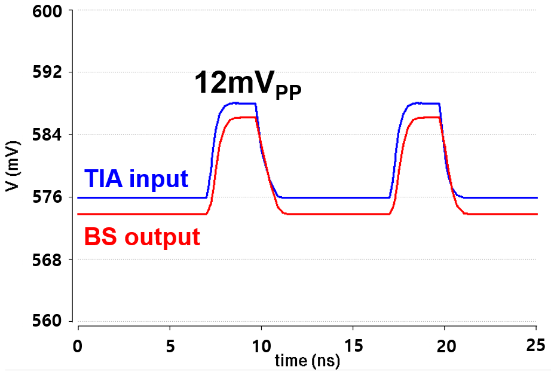## III. Measurement Results

Test chips of the proposed BFD-TIA were implemented by using a 0.13-${μ}$m CMOS process. Fig. 7 shows the chip microphotograph, where the chip core occupies the area of 0.022 mm$^{2}$. A PIN-photodiode, as an optical detector, was emulated by its electrical lumped-model with a 25-${Ω}$ resistor and a 500-fF parasitic capacitance.

Fig. 8 shows the test setup and its PCB module for measurements.

Fig. 9 demonstrates the measured output eye-diagrams of the BFD-TIA, where the output signals provide clean eye-openings for both 20 ${μ}$A$_{\mathrm{pp}}$ and 110~${μ}$A$_{\mathrm{pp}}$ input currents at different data rates.

Fig. 9. Measured eye-diagrams of the BFD-TIA for (a) 20~${μ}$A$_{\mathrm{pp}}$, (b) 110 ${μ}$A$_{\mathrm{pp}}$ 2$^{31}$-1 PRBS inputs.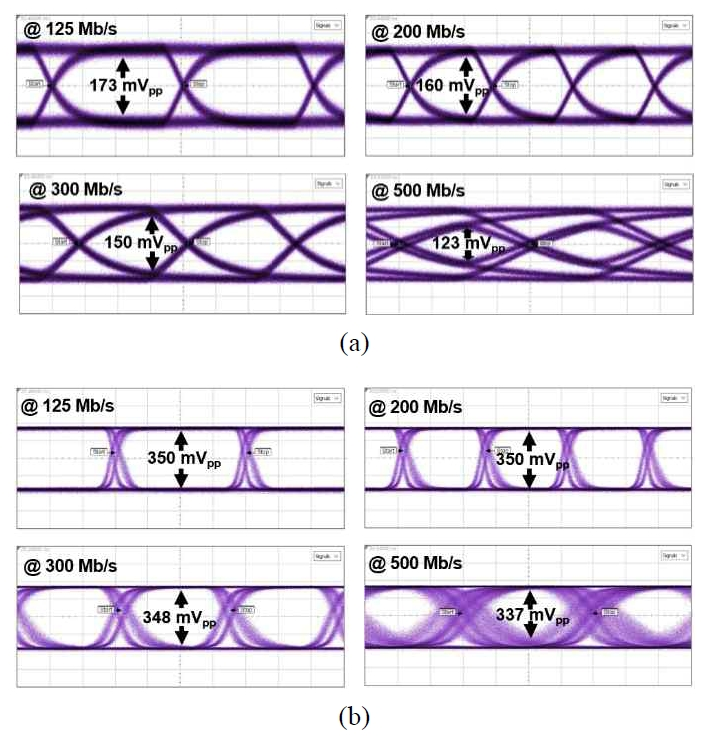Fig. 10 depicts the measured differential output eye-diagrams for 50 ${μ}$A$_{\mathrm{pp}}$ 2$^{31}$-1 PRBS inputs, demonstrating less than 3% mismatch of the differential eye-openings at two different data rates of 125 Mb/s and 300 Mb/s.

Fig. 10. Measured differential output eye-diagrams of the BFD-TIA with 50 ${μ}$A$_{\mathrm{pp}}$ 2$^{31}$-1 PRBS inputs at different data rates of (a) 125 Mb/s, (b) 300 Mb/s.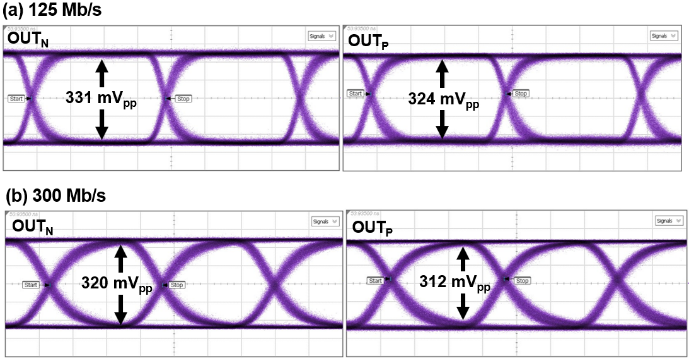Fig. 11 shows the test setup for pulse measurements.

Fig. 12 demonstrates the output pulses of the BS circuit and the BFD-TIA for 20~${μ}$A$_{\mathrm{pp}}$ input pulses, indicating that the input voltage(v$_{\mathrm{in}}$) of the BFD-TIA is estimated to 21.2~mV$_{\mathrm{pp}}$ by dividing the output voltage(OUT$_{\mathrm{P}}$ = 336~mV$_{\mathrm{pp}}$) by the voltage gain(= 24 dB). Then, it is very similar to the BS output voltage of 20.4 mV$_{\mathrm{pp}}$, leading to 3.8-% mismatch.

Fig. 12. Measured output pulses of both the BS and the BFD-TIA with 20 ${μ}$A$_{\mathrm{pp}}$ input pulses.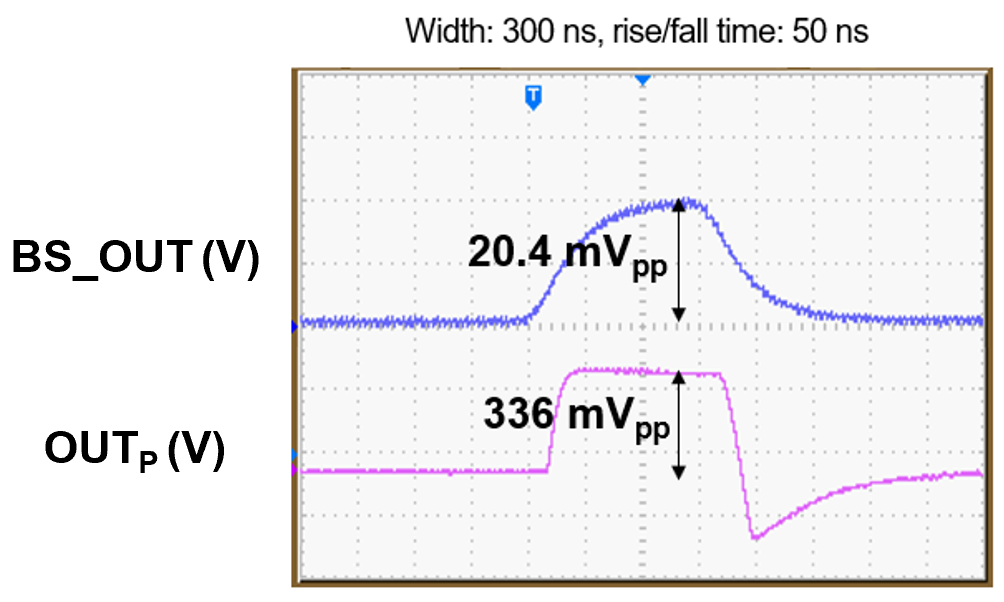Therefore, it confirms that the photodiode capacitance(C$_{\mathrm{pd}}$) can be effectively eliminated by rendering the voltage across the photodiode equal, hence reducing the deterioration of the overall performance such as bandwidth and noise.

Table 1 compares the performance of the proposed BFD-TIA with the previously reported prior arts. When compared to the BiCMOS inverter TIA in (4), the BFD-TIA provides 39% larger transimpedance gain, 14% less noise, and 89% less power consumption. Comparison with the single-ended VCF TIA in (5) reveals that the BFD-TIA provides 11.3% higher transimpedance gain and 19.5% lower power consumption for a little higher noise current spectral density. Although the capacitive feedback TIA in (6) shows much better noise performance with variable transimpedance gain than our BFD-TIA, it dissipates 70% larger power. When compared to the CMOS inverter TIA in (7), the BFD-TIA yields 9.3% higher transimpedance gain and 79% lower power dissipation. In particular, the TIAs reported in (5,6) were pseudo-differential because their single-ended input signals passed through either a low-pass filter(LPF) or a replica circuit to form differential outputs.

Table 1. Performance comparison of the proposed BFD-TIA with previously reported prior arts

 Parameters     this work CMOS technology (μm) 0.35 BiCMOS 0.18 0.35 0.13 0.13 Input configuration INV VCF CF INV BFD Circuit topology Single-ended Single-ended Single-ended Single-ended Differential Photodiode cap. (pF) 1.5 0.5 2.5~5 2 0.5 Transimpedance gain (dBΩ) 52.5 76.3 78~110 78 86 Operation Speed (Mb/s) 300* 800 260* 830* 500 Noise current spectral density $(\mathrm{pA}/\sqrt{\mathrm{Hz}})$) 9.2 6.3 1.36 5.6 7.5 Power supply rejection ratio (dB) N/A N/A N/A N/A 23 Power dissipation per channel (mW) 220 29.8 79 114 24 FoM (pJ/b) 733 37.3 304 137 48 Core area (mm$^2$) 5.86 (w/ TDC) 5.5 (16-channel) 1.2 0.59 0.022

INV: inverter, VCF: voltage-mode CMOS feedforward, CF: capacitive feedback, TDC: time-to-digital converter

* Estimated operation-speed, ** Figure of merit(FoM) =$\frac{\textit{Powerdiss}.\left(mW\right)}{\textit{Operation}~ \textit{Speed}\left(Mb/s\right)}$

However, the proposed BFD-TIA provides differential structure from the input stage by exploiting the MC input stage with no need of either a low-pass filter or a replica, hence showing good PSRR performance and smaller chip area. Finally, we have utilized the well-known figure-of-merit(FoM) that is defined by the ratio of the power dissipation over the operation speed, demonstrating that the BFD-TIA provides superior FoM characteristics among the TIAs together with (5).

## IV. CONCLUSIONS

In this paper, we have realized a fully differential TIA in a 0.13-${μ}$m CMOS process that exploits a novel mirrored-cascode input configuration to generate differential signaling even from the input stage. Also, bootstrapping circuit technique is applied in the input stage and enables to isolate the photodiode capacitance from the noise and bandwidth determination by generating the same AC voltage as the input-node voltage and then connecting it onto the cathode of the photodiode. Hence, it can be concluded that the proposed BFD-TIA provides a potential for low-power and low-noise solutions in LiDAR systems for the applications of unmanned autonomous vehicles as well as in the area of near-field image processing systems.

### ACKNOWLEDGMENTS

This research was supported by the MSIT(Ministry of Science and ICT), Korea, under the ITRC(Information Technology Research Center) support program(IITP-2020-2018-0-01421) supervised by the IITP(Institute for Information & communications Technology Promotion).

### REFERENCES

1
Buchbinder E., Speed Detection: LADAR, www.ee.eng.buffalo.edu/faculty/cartwright/teaching/ ee494s99/presentations/lasar.pdf.2
Kurtti S., Jansson J. –P., Kostamovaara J., Early Access in 2019, A CMOS Receiver-TDC Chip Set for Accurate Pulsed TOF Laser Ranging, IEEE Trans. on Instr. and Meas.3
Kim S. G., Hong C., Eo Y., Kim J. –H., Park S. M., 2019, A 40-GHz Mirrored-Cascode Differential TIA in 65nm CMOS, IEEE J. of Solid-State Circuits, Vol. 54, No. 5, pp. 1468-14744
Kurtti S., Kostamovaara J., Mar 2009, Laser radar receiver channel with timing detector on front end unipolar-to-bipolar pulse shaping, IEEE J. Solid-State Circuits, Vol. 44, No. 3, pp. 835-8475
Hong C., Kim S. –H., Kim J. –H., Park S. M., Sep 2018, A linear-mode LiDAR sensor using a multi-channel CMOS TIA array, IEEE Sensors J., Vol. 18, No. 17, pp. 7032-70406
Cho H. S., Kim C. –H., Lee S. –G., Oct 2014, A High-Sensitivity and Low-Walk Error LADAR Receiver for Military Application, IEEE Tran. Circuits and Systems I, Vol. 61, No. 10, pp. 3007-30157
Ngo T.–H., et al , Feb 2013, Wideband Receiver for a Three-Dimensional Ranging LADAR System, IEEE Tran. Circuits and Sys. I, Vol. 60, No. 2, pp. 448-456## Author

Yoonji Park received the B.S. degree in electronics engineering from Ewha Womans University, Seoul, South Korea, in 2018.

She is currently working toward the M.S. degree at the same university.

Her current research interests include CMOS analog integrated circuits and architectures for optical interconnects and LiDAR systems.

Ji-Hoon Kim received the B.S. (summa cum laude) and Ph.D. degrees in electrical engineering and computer science from KAIST, Daejeon, South Korea, in 2004 and 2009, respectively.

In 2009, he joined Samsung Electronics. In 2018, he joined the faculty of the department of electronic and electrical engineering, Ewha Womans University, where he is currently an associate professor.

His current interests include CPU/DSP, communication modem, and low-power SoC design for security/biomedical systems.

Dr. Kim is a technical committee member of the circuits and systems for communications and VLSI systems and applications in the IEEE Circuits and Systems Society.

He was a recipient of the best design award at Dongbu HiTek IP Design Contest in 2007 and first place award at the International SoC Design Conference Chip Design Contest in 2008.

Sung Min Park received the B.S. degree in electrical and electronic engineering from KAIST, Korea, in 1993.

He received the M.S. degree in electrical engineering from University College London, U.K., in 1994, and the Ph.D. degree in electrical and electronic engineering from Imperial College London, U.K., in May 2000.

In 2004, he joined the faculty of the Department of Electronics Engineering at Ewha Womans University, Seoul, Korea, where he is currently a Professor.

His research interests include highspeed analog/digital integrated circuit designs in submicron CMOS and SiGe HBT technologies for the applications of optical interconnects, silicon photonics, and RF communications.

Prof. Park has served on the technical program committees of a number of international conferences including ISSCC (2004–2009).